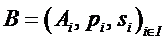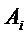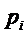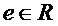Mathematical Models of Games of Chance and Gambling
 Problem Gambling |  Home | Gambling | Articles | Books

Models and their functions
Games of chance are developed in their physical consumer-ready form on the basis of mathematical models, which stand as the premises of their existence and represent their physical processes. As an example (of physical-process representation), roulette betting is represented as follows:
A roulette bet is defined as a finite family, whereis a subset of the set R of roulette numbers, which allows a single placement according to the configuration of the roulette table (such as for straight, split, corner column, color bet, etc.),is the payout of, andis the stake of the placement. All bets B so defined form the bet space. Then, for each bet B, a profit functionis defined as follows:R,, where R is the set of real numbers andis the characteristic function of set A. Variablestands for the outcome of a game. The value of functionis called the profit of bet B (Barboianu, 2007). For each bet B, functionmay take negative or non-negative values. The profit function expresses the net amount the player wins or loses after the spin as a result of the player’s bet (applying the convention that profit can also be negative, that is, a loss). This formal system is part of a mathematical model of the roulette game (there is more than one model involved in the game of roulette) representing some of the physical processes of betting, namely chip placements and their overall financial result after the spin. Using the mathematical structure of the bet space and the properties of the profit function, a relation of equivalence between bets can be defined; in the real world, the relation represents a natural financial equivalence between bets. The inferred properties of the equivalence of the bets in the mathematical model yield criteria of optimization of roulette betting with respect to personal money and time management. This mathematical model of a roulette bet can be generalized and adapted further to other types of bets specific to other games.
Mathematical models can additionally represent gambling systems related to several plays, several games, and generally any quantifiable gambling activity. For instance, still from roulette, the Martingale system (keeping the same bet and raising its stake with the same multiplier successively until the first success) is modeled through a geometrical progression whose partial sums of terms obey a certain inequality, which in fact ensure an overall positive profit when a bet is won. In the Martingale example, not only do we have a representation of a physical process (the described betting system) through a mathematical model, but also a mathematical explanation (through the same model) of the trust this roulette system is granted by gamblers.

Two types of models
Games of chance and gambling as a quantifiable activity are represented in applied mathematics through specific mathematical models which can be distinguished through two main categories with respect to the purposes they serve, which I call:
1.     Probabilistic and statistical models
2.     Functional models
While probabilistic and statistical models serve for the applications related to the games’ outcomes occurring under conditions of uncertainty, functional models serve to represent the physical systems and processes that make the games actually function as well as for applications related to the functioning.
As examples, computing the probability of hitting a specific number or at least a specific quantity of winning numbers at a specific lottery is workable within a probabilistic model, which assumes establishing the right probability field within which to work with the appropriate discrete probability distribution. Computing statistical means and errors (in the statistical sense) is workable within a statistical model by establishing the distribution of the random variable which describes the outcome and using mathematical means and measures such as expected value, deviation, dispersion, or variance. Card movement in poker is described through a functional model dealing with combinations of symbols and values specific to cards. Roulette complex bets are represented in a functional model as elements of a mathematical structure with vectorial and topological features. Paylines of a multiline slot machine are represented as lines in a Cartesian grid or paths in a graph, and their mutual independence is described through topological properties, all these still within a functional model (Barboianu, 2013a).
Below are the purposes and mathematical governing theories involved in each of the two categories of mathematical models used in gambling.
1. Probabilistic and statistical models. Purposes: quantification of the gambling uncertainty, measuring risk and possibility, prediction, computation of the parameters characterizing games, of the means and statistical errors, providing practical statistics (collections of data), optimization, providing strategy and optimal play. Governing theories: Measure Theory, Probability Theory, Mathematical Statistics, Real Analysis, Decision Theory.
2. Functional models. Purposes: description of the gaming processes and of the functioning of the games, optimization, providing strategy and optimal play, providing the necessary theoretical support for the probabilistic and statistical models. Governing theories: Set Theory, Combinatorics, Number Theory, Algebra, Topology, Geometry, Graph Theory, Real Analysis.
A game and the gambling activity related to that game are represented by several mathematical models, each of them serving a purpose for the applied mathematician. This multimodel feature holds even for the same subcategory of models. For instance, computing the strength of a poker hand in terms of probabilities assumes establishing several different probability fields for the events related to one’s own hand and those events of type “at least one” related to the opponents’ hands, which means a different probabilistic model for each application. Every game of chance is represented by models from both the first and second categories, even though a model from the latter category may be trivial. This happens because outcomes and uncertainty are specific to games of chance by definition (thus explaining the existence of first-category models) and every probabilistic/statistical model needs a functional model in order to ensure the grounding mathematical structures necessary for the governing theories of the probabilistic/statistical model to be applied. For example, any probability computation within a probabilistic model needs a priori a grounding model representing the gaming events to be measured, which must belong to a Boolean structure, and this latter model is a functional one.

Prevalence of the probabilistic and statistical models
There is a prevalence of models of the first category in the interest of all parties involved in the study of gambling – researchers, game producers and operators, and players. This prevalence is explainable first through the fact that these models provide measures, estimations, and predictions for the financial results of the gambling activity, which in turn generate the most important indicators for the commercial aspect of the phenomenon. These models actually provide game producers and operators with a mathematical “guarantee” that a certain game can be run with no risk of ruin for the house over the long run; for the players, probabilities and statistical indicators are the most important criteria in making gaming decisions. Second, games of chance have simple processes of functioning. For commercial reasons, they are designed to be as undemanding as possible with straightforward sets of rules and short timeframes of the sessions; according to this uncomplicated design, the functional models representing them are usually simple, sometimes trivial (unlike other types of games – for instance strategy games such as chess – whose complexity is modeled through richer mathematical models). There are also exceptions to this functional-model-simplicity rule –  that is, games whose apparently simple functioning hides complex mathematical models. Such is the case with roulette betting, in which complex bets are represented as elements of a mathematical structure generating vectorial and topological spaces and classes of equivalence within which various further applications can be developed. This exception applies also to multiline slots, where probability applications related to groups of paylines rely on a representation of the display as part of a discrete mathematical structure (Cartesian grid or graph) generating a metric space (and implicitly a topology), within which properties such as connection, neighboring, and independence are defined to serve the probabilistic models (Barboianu, 2013a, 2013b).
This prevalence of the models of the first category in the study of gambling is also reflected in the content of the existent courses of gambling mathematics – either experimental or school based – where gambling is presented exclusively as a plain, direct application of Probability Theory and Mathematical Statistics.

Epistemic view of the act of mathematical modeling
Mathematical modeling is the main mean of inference in science, and modern to contemporary accounts of the role of mathematics in scientific explanation of physical phenomena have argued for its indispensability (see Quine, 1981; Colyvan, 1998; Baker, 2009; Saatsi, 2011). The inference based on mathematical models, which is the core method of scientific reasoning, is possible first because mathematics is a rich source of structures, and second because of the representation function of a mathematical model, which allows mathematical structures to be recognized as embedded in the physical world. Here is how such inference works in brief, in terms of Bueno and Colyvan’s (2011) inferential conception of mathematical modeling:
Step 1. A mapping is established from the investigated physical system to a convenient mathematical structure, a step called immersion. The purpose is to relate the relevant aspects of the physical system (which is thus idealized) with the appropriate mathematical theory.
Step 2. Consequences are drawn from the formal systems within the mathematical theory that deals with those systems (the governing theory), through logical inference. This step is called derivation.
Step 3. A mapping is established from the mathematical structure to the initial physical system (not necessarily the inverse of the immersion mapping), and the mathematical consequences that were obtained in the derivation step are interpreted in terms of the initial (non-idealized) physical system. This step is called interpretation.Figure. The inferential conception of applied mathematics

The mapping account (also called structural account) establishes homomorphic or isomorphic relations between mathematical structures from within a mathematical theory and mathematical structures recognized in the idealized physical system (Bueno & French, 2012). This feature of the mapping account justifies epistemically the inference based on the mathematical model. The practical execution of the three steps above is the object of applied mathematics, and the setup of the mappings from steps 1 and 3 is called mathematical modeling.
There are also other accounts of the application of mathematics in physical universe, including Frege’s (1884, 1889) semantic account and Pincock’s (2004) internal-relation account. Yet, the structural account is the most representative in the case of gambling, as well as in the most of the domains and sciences where the mathematical apparatus is required.

References:

Baker, A. (2009). Mathematical Explanation in Science. British Journal for the Philosophy of Science, 60(3), pp. 611-633.

Barboianu, C. (2007). Complex bets. In Roulette Odds and Profits: The Mathematics of Complex Bets (pp. 24–30). Craiova, Romania: Infarom.

Barboianu C., (2013a). Configuration of the display. In The mathematics of slots: Configurations, combinations, probabilities (pp. 18–36). Craiova, Romania: Infarom.

Barboianu C., (2013b). The probabilities of the winning events related to several lines. In The mathematics of slots: Configurations, combinations, probabilities (pp. 107–125). Craiova, Romania: Infarom.

Bueno, O. & Colyvan, M., 2011. An Inferential Conception of the Application of Mathematics. Noûs, 45(2), pp. 345-374.

Bueno, O. & French, S., 2012. Can Mathematics Explain Physical Phenomena? The British Journal for the Philosophy of Science, 63(1), pp. 85-113.

Colyvan, M. (1998). In Defence of Indispensability, Philosophia Mathematica, 6(1), pp. 39–62.

Frege, G., 1884. Die Grundlagen der Arithmetik: eine logisch-mathematische Untersuchung über den Begriff der Zahl, Breslau: W. Koebner. Translated as The Foundations of Arithmetic: A logico-mathematical enquiry into the concept of number, by J.L. Austin, Oxford: Blackwell, second revised edition, 1974.

Frege, G., 1889. Begriffsschrift, eine der arithmetischen nachgebildete Formelsprache des reinen Denkens, Halle a. S.: Louis Nebert. Translated as Concept Script, a formal language of pure thought modelled upon that of arithmetic, by S. Bauer-Mengelberg in J. vanHeijenoort (ed.), From Frege to Gödel: A Source Book in Mathematical Logic, 1879–1931, Cambridge, MA: Harvard University Press, 1967.

Pincock, C., 2004. A New Perspective on the Problem of Applying Mathematics. Philosophia Mathematica, 12(3), pp. 135-161.

Quine, W.V. (1981). Things and Their Place in Theories. In Theories and Things, (pp.1-23). Cambridge: Harvard University Press.

Saatsi, J. (2011). The Enhanced Indispensability Argument: Representational versus Explanatory Role of Mathematics in Science. British Journal for the Philosophy of Science, 62(1), 143-154

This entry should be cited as:
Barboianu, C. (2014). Mathematical Models of Games of Chance and Gambling. Retrieved from http://probability.infarom.ro/models.html .

back to Problem Gambling index

 Author The author of this page is Catalin Barboianu (PhD). Catalin is a games mathematician and problem gambling researcher, science writer and consultant for the mathematical aspects of gambling for the gaming industry and problem-gambling institutions. Profiles:   Linkedin   Google Scholar   Researchgate» Main menu Mathematical models Informative-ethical contributions Didactical-cognitive contributions Clinical-cognitive contributions

 Problem-gambling research centers NCRG OPGRC

 Problem-gambling clinical centers GTC CAMH ICYGPHRB MMPGRTC